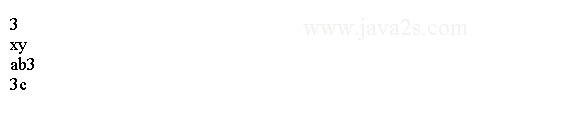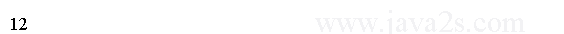# OCA Java SE 8 Core Java APIs - Java String

A string is basically a sequence of characters; here's an example:

```String name = "java2s.com";
```

String type is an example of a reference type.

The reference types are created using the new keyword.

In Java, the following two lines both create a String:

```String name = "java2s.com";
String name = new String("java2s.com");
```

Both give you a reference variable of type name pointing to the String object "java2s.com".

String class is special and doesn't need to be instantiated with new.

## Concatenation

"1" + "2" becomes "12" since Java combines the two String objects.

Placing one String before the other String and combining them together is called string concatenation.

+ operator can be used in two ways within the same line of code.

• If both operands are numeric, + means numeric addition.
• If either operand is a String, + means concatenation.
• The expression is evaluated left to right.
```public class Main{
public static void main(String[] argv){
System.out.println(1 + 2);           // 3
System.out.println("x" + "y");       // xy
System.out.println("a" + "b" + 3);   // ab3
System.out.println(1 + 2 + "c");     // 3c
}
}
```

The code above generates the following result.The first example uses the first rule. Both operands are numbers, so we use normal addition.

The second example is string concatenation described in the second rule.

The third example combines both the second and third rules. We start on the left, Java evaluates "a" + "b". Then Java looks at the remaining expression of "ab" + 3.

The fourth example starts with the third rule and consider 1 + 2 in the first around. Then we have 3 + "c" and use the second rule to have "3c".

```int three = 3;
String four = "4";
System.out.println(1 + 2 + three + four);
```

s += "2" means the same thing as s = s + "2".

```public class Main{
public static void main(String[] argv){
String s = "1";             // s currently holds "1"
s += "2";                   // s currently holds "12"
System.out.println(s);
s += 3;                     // s currently holds "123"
System.out.println(s);      // 123

}
}
```

The code above generates the following result.## Note

Once a String object is created, we cannot change it any more. String is immutable.

```public class Main{
public static void main(String[] argv){
String s1 = "1";
/*from  w ww.j av a2  s  .  co  m*/
String s2 = s1.concat("2");

s2.concat("3");

System.out.println(s2);
}
}
```

The following code reassign the value back to s2.

```String s2 = s1.concat("2");
```

While the following code won't change s2

```s2.concat("3");
```

The code above generates the following result.## String Pool

The string pool in the Java virtual machine (JVM) collects all these strings.

The string pool contains literal values that appear in your program.

For example, "java2s.com" is a literal and therefore goes into the string pool.

myObject.toString() returns a string but not a literal, so it does not go into the string pool.

Strings not in the string pool are garbage collected just like any other object.

Remember back when we said these two lines are subtly different?

```String name = "java2s.com";
String name = new String("java2s.com");
```

The first uses the string pool normally.

The second doesn't use the string pool and creates a new object.